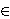### Maja Fošner, Benjamin Marcen, Nejc Širovnik and Joso Vukman

Faculty of Logistics, University of Maribor, Mariborska cesta 7, 3000 Celje, Slovenia
e-mail: maja.fosner@fl.uni-mb.si

Faculty of Logistics, University of Maribor, Mariborska cesta 7, 3000 Celje, Slovenia
e-mail: benjamin.marcen@fl.uni-mb.si

Faculty of Natural Sciences and Mathematics, University of Maribor, Koroška cesta 160, 2000 Maribor, Slovenia
e-mail: nejc.sirovnik@uni-mb.si

Faculty of Mathematics, Natural Sciences and Information Technologies , University of Primorska, Glagoljaška 8, 6000 Koper
and
Institute of Mathematics, Physics and Mechanics, Jadranska 19, 1000 Ljubljana, Slovenia
e-mail: joso.vukman@uni-mb.si, joso.vukman@gmail.com

Abstract.   In this paper we prove the following result. Let n≥ 1 be some fixed integer and let R be a prime ring with 2n < char(R) ≠ 2. Suppose there exist additive mappings S,T:R → R satisfying the relations

S(x2n)=S(x)x2n-1+xT(x)x2n-2+x2S(x)x2n-3+ ⋯ +x2n-1T(x),

T(x2n)=T(x)x2n-1+xS(x)x2n-2+ x2T(x)x2n-3+ ⋯ +x2n-1S(x)

for all xR. In this case S and T are of the form 2S(x)=D(x)+ζ (x), 2T(x)=D(x)-ζ (x) for all xR, where D:R → R is a derivation and ζ is an additive mapping, which maps R into its extended centroid. Besides, ζ (x2n)=0 for all xR. Functional equations related to bicircular projections are also investigated.

2010 Mathematics Subject Classification.   16R60, 16W10, 39B05.

Key words and phrases.   Derivation, functional identity, bicircular projection.

Full text (PDF) (access from subscribing institutions only)

DOI: 10.3336/gm.49.2.06

References:

1. K. I. Beidar, W. S. Martindale 3rd and A. V. Mikhalev, Rings with generalized identities, Marcel Dekker, Inc., New York, (1996).
MathSciNet

2. K. I. Beidaar and Y.-Fong, On additive isomorphisms of prime rings preserving polynomials, J. Algebra 217 (1999), 650-667.
MathSciNet     CrossRef

3. K. I. Beidar, M. Brešar, M. A. Chebotar and W. S. Martindale 3rd, On Herstein's Lie map Conjectures II, J. Algebra 238 (2001), 239-264.
MathSciNet     CrossRef

4. K. I. Beidar, A. V. Mikhalev and M. A. Chebotar, Functional identities in rings and their applications, Russian Math. Surveys 59 (2004), 403-428.
MathSciNet     CrossRef

5. F. Botelho and J. Jamison, Generalized bicircular projections on minimal ideals of operators, Proc. Amer. Math. Soc. 136 (2008), 1397-1402.
MathSciNet     CrossRef

6. F. Botelho and J. Jamison, Generalized bi-circular projections on spaces of analytic functions, Acta Sci. Math. (Szeged) 75 (2009), 527-546.
MathSciNet

7. M. Brešar, M. A. Chebotar and W. S. Martindale 3rd, Functional identities, Birkhäuser Verlag, Basel-Boston-Berlin (2007).
MathSciNet

8. J. Cusack, Jordan derivations on rings, Proc. Amer. Math. Soc. 53 (1975), 321-324.
MathSciNet     CrossRef

9. A. Fošner and J. Vukman, Some functional equations on standard operator algebras, Acta Math. Hungar. 118 (2008), 299-306.
MathSciNet     CrossRef

10. A. Fošner and D. Ilišević, Generalized bicircular projections via rank preserving maps on the spaces of symmetric and antisymmetric operators, Oper. Matrices 5 (2011), 239-260.
MathSciNet     CrossRef

11. M. Fošner and D. Ilišević, On a class of projections on *-rings, Comm. Algebra 33 (2005), 3293-3310.
MathSciNet     CrossRef

12. M. Fošner, D. Ilišević and C.-K. Li, G-invariant norms and bicircular projections, Linear Algebra Appl. 420 (2007), 596-608.
MathSciNet     CrossRef

13. M. Fošner and J. Vukman, On some equations in prime rings, Monatsh. Math. 152 (2007), 135-150.
MathSciNet     CrossRef

14. M. Fošner and J. Vukman, On some functional equations in rings, Commun. Algebra 39 (2011), 2647-2658.
MathSciNet     CrossRef

15. I. N. Herstein, Jordan derivations of prime rings, Proc. Amer. Math. Soc. 8 (1957), 1104-1110.
MathSciNet     CrossRef

16. D. Ilišević, Generalized bicircular projections via the operator equation α X2+AY2+β XAY+A=0, Linear Algebra Appl. 429 (2008), 2025-2029.
MathSciNet     CrossRef

17. D. Ilišević, Generalized bicircular projections on JB* -triples, Linear Algebra Appl. 432 (2010), 1267-1276.
MathSciNet     CrossRef

18. J. Jamison, Generalized bicircular projections on spaces of operator an JB* -triples, Rocky Mountain. J. Math. 41 (2011), 1241-1245.
MathSciNet     CrossRef

19. I. Kosi-Ulbl and J. Vukman, A note on derivations in semiprime rings, Int. J. Math. & Math. Sci. 20 (2005), 3347-3350.
MathSciNet     CrossRef

20. I. Kosi-Ulbl and J. Vukman, On some functional equations on standard operator algebras, Glas. Mat. Ser. III 44(64) (2009), 447-455.
MathSciNet     CrossRef

21. L. H. Rowen, Some results on the center of a ring with polynomial identity, Bull. Amer. Math. Soc. 79 (1973), 219-223.
MathSciNet     CrossRef

22. L. L. Stachó and B. Zalar, Bicircular projections on some matrix and operator spaces, Linear Algebra Appl. 384 (2004), 9-20.
MathSciNet     CrossRef

23. L. L. Stachó and B. Zalar, Bicircular projections and characterization of Hilbert spaces, Proc. Amer. Math. Soc. 132 (2004), 3019-3025.
MathSciNet     CrossRef

24. J. Vukman, On functional equations related to bicircular projections, Glas. Mat. Ser. III 41(61) (2006), 51-55.
MathSciNet     CrossRef